# NCERT Solutions for Class 8 Maths Chapter 10 Visualising Solid Shapes

Exercise 10.1 Page No: 157

1. For each of the given solid, the two views are given. Match for each solid the corresponding top and front views. The first one is done for you.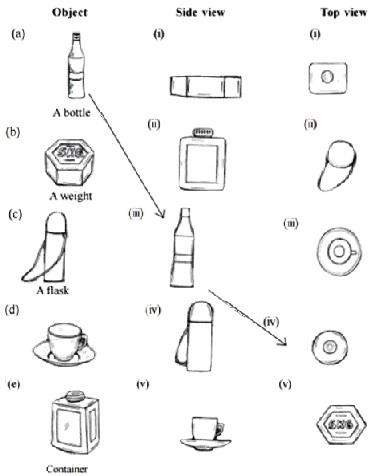Solution:

(a) (iii) (iv)

(b) (i) (v)

(c) (iv) (ii)

(d) (v) (iii)

(e) (ii) (i)

2. For each of the given solid, the three views are given. Identify for each solid the corresponding top, front and side views.Solution:

(a) (i) Front (ii) Side (iii) Top view

(b) (i) Side (ii) Front (iii) Top view

(c) (i) Front (ii) Side (iii) Top view

(d) (i) Front (ii) Side (iii) Top view

3. For each given solid, identify the top view, front view and side view.Solution:

(a) (i) Top view (ii) Front view (iii) Side view

(b) (i) Side view (ii) Front view (iii) Top view

(c) (i) Top view (ii) Side view (iii) Front view

(d) (i) Side view (ii) Front View (iii) Top view

(e) (i) Front view (ii) Top view (iii) Side view

4. Draw the front view, side view and top view of the given objects:Solution: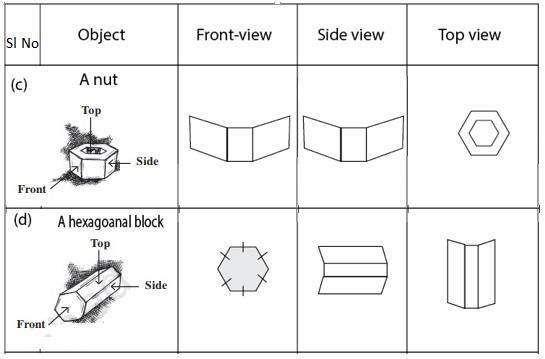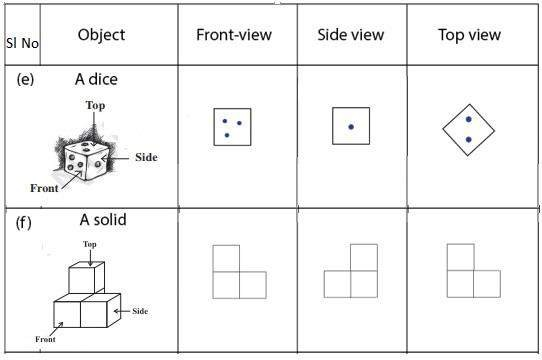Exercise 10.2 Page No: 157

1. Look at the given map of a city.Colour the map as follows: Blue-water, red-fire station , orange-library, yellow – schools, Green – Park , Pink – College, Purple – Hospital , Brown-Cemetery.

(a) Mark a green ‘X’ at the intersection of Road C and Nehru Road, Green ‘Y’ at the intersection of Gandhi Road and Road A.

(b) In red, draw a short street route from library to the bus depot.

(c) Which is the further east, the city park or the market?

(d) Which is further south, the primary School or the Sr. Secondary School?

Solution:

(a)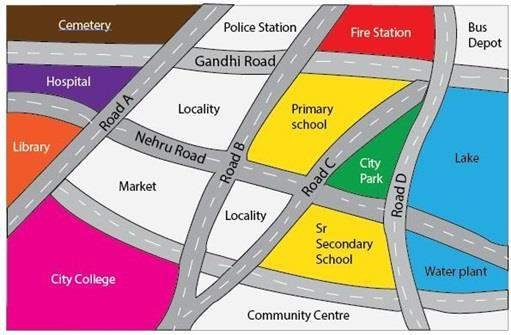(b)(c)(d) City Park

(e) Sr. Secondary School

2. Draw a map of your class room using proper scale and symbols for different objects.

Solution:

Can be anything. For your reference we have shared one classroom picture:3. Draw a map of your school compound using proper scale and symbols for various features like playground, main building garden etc.

Solution:

Do it yourself

4. Draw a map giving instructions to your friends so that she reaches your house without any difficulty.

Solution:

Do it yourself

Exercise 10.3 Page No: 166

1. Can a polyhedron have for its faces:

(i) 3 Triangles?

(ii) 4 triangles?

(iii) A square and four triangles?

Solution:

(i) No, such polyhedrons are not possible. Such figures should have minimum 4 faces.

(ii) Yes, a triangular pyramid has 4 triangular faces.

(iii) Yes, as square pyramid has a square face and 4 triangular faces.

2. Is it possible to have a polyhedron with any given number of faces? (Hint: Think of a pyramid)

Solution:

It is possible, only if the number of faces is greater than or equal to 4.

3. Which are prisms among the following: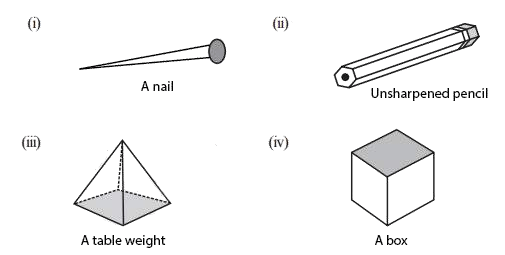Solution:

(i) A nail: Not a polyhedron as it has a curved surface. This is not a prism.

(ii) Unsharpened Pencil: It is a prism.

(iii) A table Weight: It is not a prism.

(iv) A Box: It is a prism.

4. (i) How are prisms and cylinders alike?

(ii) How are pyramids and cones alike?

Solution:

(i) A cylinder can be looks like circular prism, a prism with circular base.

(ii) A cone can be a circular pyramid, a pyramid with circular base.

5. Is a square prism same as a cube? Explain.

Solution:

Yes, a square prism can also be a cube. A square prism has a square as its base. However, its height is not necessarily same as the side of the square. Thus, a square prism can also be a cuboid.

6. Verify Euler’s formula for these solids.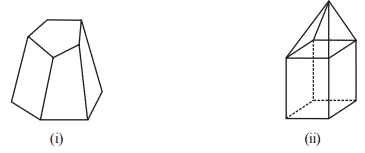Solution:

(i) Number of faces, F = 7

Number of edges, E = 15

Number of vertices, V = 10

As per formula, F + V – E = 2

Substitute the values, we have

F + V – E = 7 + 10 – 15

= 2

Hence, verified.

(ii) Here, F = 9, V = 9 and E = 16

Using formula, F+ V – E = 2

F + V – E = 9 + 9 – 16 = 2

Hence, Euler’s formula is verified.

7. Using Euler’s formula, find the unknown:

 Faces ? 5 20 Vertices 6 ? 12 Edges 12 9 ?

Solution:

Euler’s formula: F + V – E = 2

Where, F = Faces, V = Vertices and E = Edges

(i) F + 6 – 12 = 2

F = 2 + 6

⇒ F = 8

(ii) 5 + V – 9 = 2

V – 4 = 2

⇒ V = 6

(iii) 20 + 12 – E = 2

32 – E = 2

⇒ E = 30

8. Can a polyhedron have 10 faces, 20 edges and 15 vertices?

Solution:

From the given data, we have

F = 10

E = 20

V = 15

Every polyhedron satisfies Euler’s formula, which is stated as, F + V – E = 2

For the given polygon,

F + V – E = 10 + 15 – 20 = 25 – 20 = 5, which is not equal to 2

Therefore, A polyhedron is not possible as Euler’s formula is not satisfied.

### Key Features of NCERT Solutions for Class 8 Maths Chapter 10 Visualising Solid Shapes

1. Easy and simple techniques are used for finding accurate solutions.
2. These solutions are designed based on the latest syllabus.
3. Solutions are prepared by subject experts.
4. NCERT Solutions helps students by providing detailed explanations in a stepwise manner.
5. NCERT Solutions are helpful for the preparation of competitive exams.

Disclaimer:

Dropped Topics – 10.1 Introduction, 10.2 Views of 3D-Shapes, 10.3 Mapping Space Around Us and 10.4 Faces, Edges and Vertices.